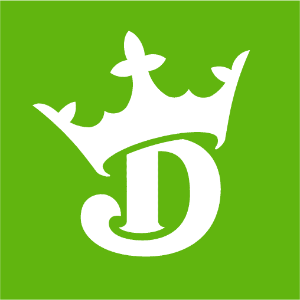DraftKings 2019 Western Conference Odds
+350
3.5 to 1Avalanche
17.6% implied probability

+350
3.5 to 1Golden Knights
17.6% implied probability

+375
3.75 to 1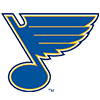Blues
16.7% implied probability

+700
7 to 1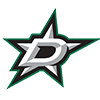Stars
9.9% implied probability

+850
8.5 to 1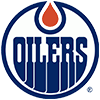Oilers
8.3% implied probability

+1000
10 to 1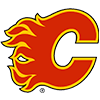Flames
7.2% implied probability

+1100
11 to 1Wild
6.6% implied probability

+2000
20 to 1Canucks
3.8% implied probability

+2100
21 to 1Predators
3.6% implied probability

+2300
23 to 1Coyotes
3.3% implied probability

+3000
30 to 1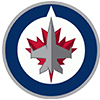Jets
2.6% implied probability

+7500
75 to 1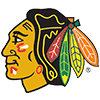Blackhawks
1.0% implied probability

+10000
100 to 1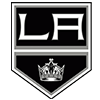Kings
0.8% implied probability

+10000
100 to 1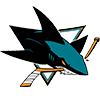Sharks
0.8% implied probability

+75000
750 to 1Ducks
0.1% implied probability